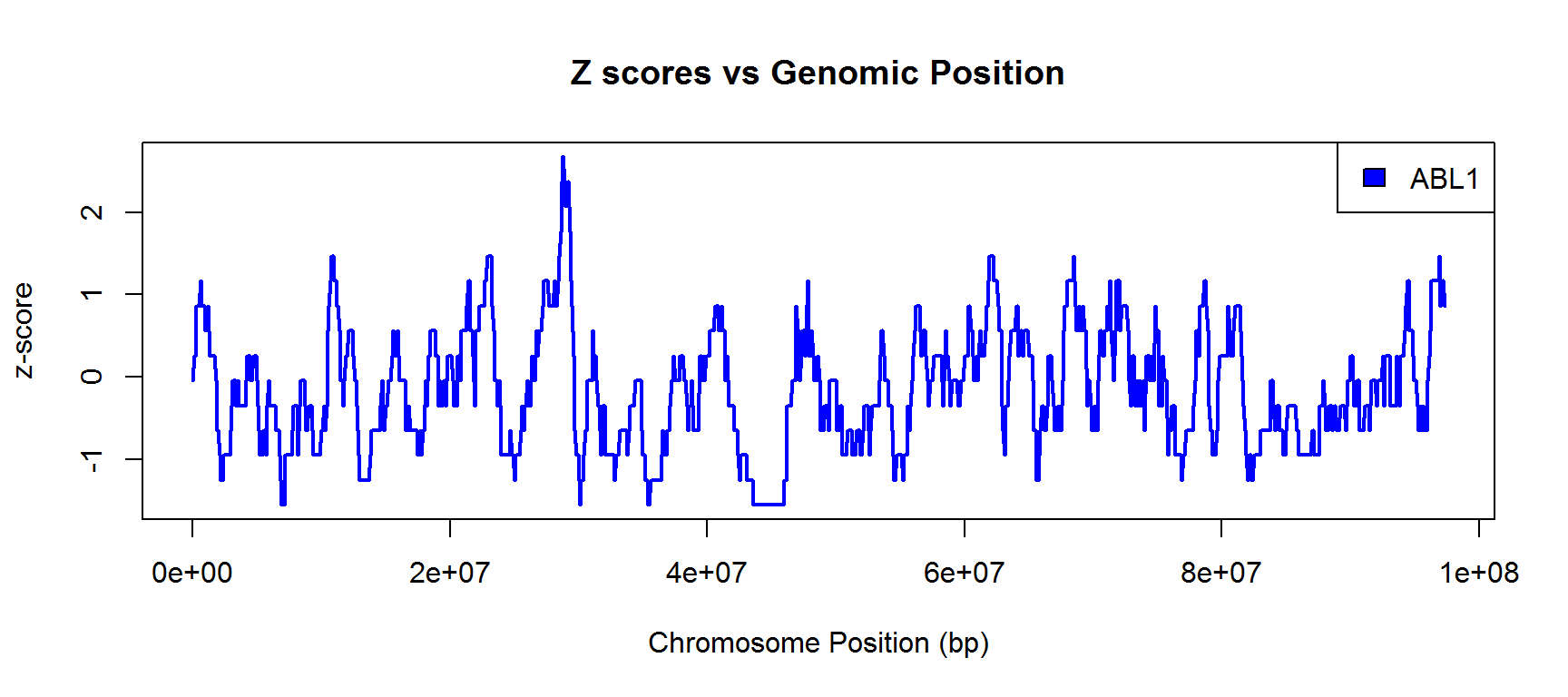# Introduction

This is an R notebook document. It allows you to mix R code, notes and outputs with nice formatting and is a nice way to record and present R analyses. You can create one of these documents in newer versions of R studio by going to File > New File > R Notebook.

I’ll use this notebook to provide worked answers to all of the exercises we did in the course.

# Introduction to R

## Exercise 1

• Use R to calculate

• 31 * 78

• 697/41

These are just simple mathematical expressions entered on the console.

31 * 78
##  2418
697 / 41
##  17
• Assign the value of 39 to x
• Assign the value of 22 to y
• Make z the value of x-y
• Display z in the console

Here we use the arrow symbols to make the assignments. They can point left or right but they must point from the data towards the variable name in which you want to store them.

You can retrieve the data stored in a variable by just entering the varible name.

39 -> x
y <- 22
x-y -> z
z
##  17
• Calculate the square root of 2345 and perform a log2 transformation on the result

Both of these operations require the use of functions. The ideal solution is to nest the two function calls together so that the call to sqrt is inside the arguments to log2.

log2(sqrt(2345))
##  5.597686

## Exercise 2

• Create a vector called vec1 containing the number 2 5 8 12 16

There is no mathematical relationship between these numbers so we need to manually create the vector using the ‘c’ function.

c(2,5,8,12,16) -> vec1
• Use x:y notation to make a second vector called vec2 containing the numbers 5 to 9

Because this is a series we can take a shortcut to make it using the language shortcut which makes integer series between two values.

5:9 -> vec2
• Subtract vec2 from vec1 and look at the result
vec1 - vec2
##  -3 -1  1  4  7

Because we have the same number of values in both vec1 and vec2 the equivalent positions will be paired up and we will see the subtraction results from the same positions, ie 2-5, 5-6, 8-7 etc.

• Use seq() to make a vector of 100 values starting at 2 and increasing by 3 each time.

We need to supply the from, by and length.out parameters to seq to create this series. As we’re using this in the next exercise we will save it into a new variable.

seq(from=2,by=3,length.out=100) -> number.series

number.series
##      2   5   8  11  14  17  20  23  26  29  32  35  38  41  44  47  50
##    53  56  59  62  65  68  71  74  77  80  83  86  89  92  95  98 101
##   104 107 110 113 116 119 122 125 128 131 134 137 140 143 146 149 152
##   155 158 161 164 167 170 173 176 179 182 185 188 191 194 197 200 203
##   206 209 212 215 218 221 224 227 230 233 236 239 242 245 248 251 254
##   257 260 263 266 269 272 275 278 281 284 287 290 293 296 299
• Extract the values at positions 5,10,15 and 20 in the vector of values you just created.
• Extract the values at positions 10 to 30

Both of these require making a selection in the vector using the [ ] notation. Inside the square brackets you put a vector of index positions, so the problem here is to create the vector of index positions.

The first one is small enough that you could make it manually with c() or as it’s a series you could use seq() to make it. It doesn’t matter which.

The second one is an integer series so we can use the 10:30 notation to make this quickly.

number.series[c(5,10,15,20)]
##  14 29 44 59
number.series[seq(from=5,to=20,by=5)]
##  14 29 44 59
number.series[10:30]
##   29 32 35 38 41 44 47 50 53 56 59 62 65 68 71 74 77 80 83 86 89

## Exercise 3

• Enter a list of colours (supplied in the question) into a vector called mouse.colour

We will use the c() function to manually make this vector. Because the data we are entering is text we need to surround each name with quotes so that R doesn’t try to treat it as a variable name.

c("purple","red","yellow","brown") -> mouse.colour
• Display the second element in the vector.

This is a simple selection with a single index position.

mouse.colour
##  "red"

Enter some numerical weight data (supplied in the question) into a vector called mouse.weight

c(23,21,18,26) -> mouse.weight
• Join the two vectors into a data frame called mouse.info containing 2 columns and 4 rows. Call the first column colour and the second one weight.

We will use the data.frame() function to do this. We don’t need to specify the number of rows / columns as these are defined by the data we use to create the data frame. The number of columns is the number of vectors we provide and the number of rows is the number of data points in each of those vectors.

If we pass the vectors to the data.frame() function as name=vector pairs then we can set the names for the columns when we create the data frame.

Remember that data.frame() will not save the result - you still need to use an arrow at the end to give it a name. You will see that the notebook formats the data frame we get as a nice looking table.

data.frame(colour=mouse.colour, weight=mouse.weight) -> mouse.info

mouse.info
##   colour weight
## 1 purple     23
## 2    red     21
## 3 yellow     18
## 4  brown     26
• Display just row 3
• Display just column 1
• Display row 4 column 1

All of these use 2 dimensional selections on the data frame. You again use the [ ] notation but this time you need to pass two vectors, the first for the rows and the second for the columns.

When we are pulling out a single column we can also use the [[ ]] notation, or ideally use dataframe$column notation as we have a named column. mouse.info[3,] ## colour weight ## 3 yellow 18 mouse.info[,1] ##  purple red yellow brown ## Levels: brown purple red yellow mouse.info[] ##  purple red yellow brown ## Levels: brown purple red yellow mouse.info$colour
##  purple red    yellow brown
## Levels: brown purple red yellow
mouse.info[4,1]
##  brown
## Levels: brown purple red yellow

The reason you see the text saying “Levels:…” after each line is because R converts character vectors to factor vectors when you create a data frame. A factor vector stores all of the different values it can store as a separate property, and this is what is shown in the levels value.

## Exercise 4

We could do this through the R studio interface using Session -> Set Working Directory -> Choose directory but in this script I will have to call setwd() directly. Because of the way notebooks work I’ll have to re-do this in every section which loads data.

setwd("O:/Training/Introduction to R/R_intro_data_files")
• Read the file “small_file.txt” into a new structure

This is a tab delimited file so we can use read.delim for this.

setwd("O:/Training/Introduction to R/R_intro_data_files")
small.file
##    Sample Length Category
## 1     x_1     45        A
## 2     x_2     82        B
## 3     x_3     81        C
## 4     x_4     56        D
## 5     x_5     96        A
## 6     x_6     85        B
## 7     x_7     65        C
## 8     x_8     96        D
## 9     x_9     60        A
## 10   x_10     62        B
## 11   x_11     80        C
## 12   x_12     63        D
## 13   x_13     50        A
## 14    y_1     64        B
## 15    y_2     43        C
## 16    y_3     98        D
## 17    y_4     78        A
## 18    y_5     53        B
## 19    y_6    100        C
## 20    y_7     79        D
## 21    y_8     84        A
## 22    y_9     68        B
## 23   y_10     99        C
## 24   y_11     65        D
## 25   y_12     55        A
## 26   y_13     98        B
## 27    z_1     56        C
## 28    z_2     83        D
## 29    z_3     81        A
## 30    z_4     69        B
## 31    z_5     50        C
## 32    z_6     72        D
## 33    z_7     54        A
## 34    z_8     56        B
## 35    z_9     87        C
## 36   z_10     84        D
## 37   z_11     80        A
## 38   z_12     68        B
## 39   z_13     95        C
## 40   z_14     93        D
• Read the file “Child_Variants.csv” into a new data structure.
setwd("O:/Training/Introduction to R/R_intro_data_files")
head(child.variants)
##   CHR    POS      dbSNP REF ALT QUAL FILTER   GENE                  HGVS
## 1   1  69270          .   A   G   16   PASS  OR4F5         c.180A>G(p.=)
## 2   1  69511 rs75062661   A   G  200   PASS  OR4F5  c.421A>G_p.Thr141Ala
## 3   1  69761          .   A   T  200   PASS  OR4F5  c.671A>T_p.Asp224Val
## 4   1  69897 rs75758884   T   C   59   PASS  OR4F5         c.807T>C(p.=)
## 5   1 877831  rs6672356   T   C  200   PASS SAMD11 c.1027T>C_p.Trp343Arg
## 6   1 881627  rs2272757   G   A  200   PASS  NOC2L        c.1843C>T(p.=)
## 1   1/1 ENST00000335137           3        4                75    SC   0
## 2   1/1 ENST00000335137          24       27                88   NSC   0
## 3   1/1 ENST00000335137           8        8               100   NSC   0
## 4   1/1 ENST00000335137           3        3               100    SC   0
## 5 10/14 ENST00000342066          10       11                90   NSC   0
## 6 16/19 ENST00000327044          52       56                92    SC   0
##   SAMPLE  FAMILY
## 1 D88849 COG0215
## 2 D88849 COG0215
## 3 D88849 COG0215
## 4 D88849 COG0215
## 5 D88849 COG0215
## 6 D88849 COG0215
• Display row 11

This is a simple selection. Becuase it’s a row we’re selecting there is no shortcut so we need to specify both rows and columns (even though the column selection is blank)

child.variants[11,]
##    CHR    POS      dbSNP REF ALT QUAL FILTER  GENE       HGVS EXON
## 11   1 889159 rs13302945   A   C  200   PASS NOC2L c.888+3T>G    .
## 11 ENST00000327044          26       29                89    SS   0 D88849
##     FAMILY
## 11 COG0215
• Calculate the mean of the column named MutantReadPercent.

Think about this in two steps. First we need to get at the data in that column. We can do that using $as we know the name of the column. Then we can pass that into the mean function. mean(child.variants$MutantReadPercent)
##  59.88219

## Exercise 5

• Find out how many rows in small_file have a length which is < 65.

For all filtering think of the problem in a structured way.

1. Extract the vector of values you want to filter against (in this case small_file$Length) 2. Apply the logical test (in this case < 65) 3. Either use the logical vector produced to filter the data using a selction, or use sum() to get the count of the hits. small.file$Length
##    45  82  81  56  96  85  65  96  60  62  80  63  50  64  43  98  78
##   53 100  79  84  68  99  65  55  98  56  83  81  69  50  72  54  56
##   87  84  80  68  95  93
small.file$Length < 65 ##  TRUE FALSE FALSE TRUE FALSE FALSE FALSE FALSE TRUE TRUE FALSE ##  TRUE TRUE TRUE TRUE FALSE FALSE TRUE FALSE FALSE FALSE FALSE ##  FALSE FALSE TRUE FALSE TRUE FALSE FALSE FALSE TRUE FALSE TRUE ##  TRUE FALSE FALSE FALSE FALSE FALSE FALSE sum(small.file$Length < 65)
##  14
• Create a filtered version of the child.variants data which includes only rows where MutantReadPercent >= 70.

This is the same 3 step problem.

1. Extract the MutantReadPercent column using the $notation 2. Perform the logical test on this vector (>=70) 3. Use this logical vector to define which rows to keep in a standard 2 dimension selection on the original data frame. To keep the output from the first two commands a sensible length I’m going to put them inside a call to the head() function. I’m increasing the number of values shown from the default (which is only 6) so you get a better feeling for what the data looks like. head(child.variants$MutantReadPercent, n=100)
##     75  88 100 100  90  92  97  95  80  89  89  81  33  90 100  90 100
##   100 100  89  82 100  90 100 100  92  93  90  85  93  94  57  31  90
##    34  51  39  44  42  56  90  26  28  93  93  95  89  77 100  54  37
##    50  38  30  42  76  47  42  27  50  45  22  35  36  38  45  34  47
##    54  59  28  91  22  66  57 100  35  34  46 100  26  60 100  44  33
##    82  25  21  83 100  85  36  84  75  52  94  88  78  83  86
head(child.variants$MutantReadPercent >= 70, n=100) ##  TRUE TRUE TRUE TRUE TRUE TRUE TRUE TRUE TRUE TRUE TRUE ##  TRUE FALSE TRUE TRUE TRUE TRUE TRUE TRUE TRUE TRUE TRUE ##  TRUE TRUE TRUE TRUE TRUE TRUE TRUE TRUE TRUE FALSE FALSE ##  TRUE FALSE FALSE FALSE FALSE FALSE FALSE TRUE FALSE FALSE TRUE ##  TRUE TRUE TRUE TRUE TRUE FALSE FALSE FALSE FALSE FALSE FALSE ##  TRUE FALSE FALSE FALSE FALSE FALSE FALSE FALSE FALSE FALSE FALSE ##  FALSE FALSE FALSE FALSE FALSE TRUE FALSE FALSE FALSE TRUE FALSE ##  FALSE FALSE TRUE FALSE FALSE TRUE FALSE FALSE TRUE FALSE FALSE ##  TRUE TRUE TRUE FALSE TRUE TRUE FALSE TRUE TRUE TRUE TRUE ##  TRUE child.variants[child.variants$MutantReadPercent >= 70,] -> child.variants.filtered

nrow(child.variants)
##  25822
nrow(child.variants.filtered)
##  9976
• From the filtered data frame we want to know the frequency with which G, A, T and C bases were mutated.

This filtration is going to use the REF column in the child.variants.filtered dataset. We’re going to need to do an equality (==) test to compare this to G, A, T and C. In this instance we only care about how many of the values are true so we can use a sum() on the logical vector to get that value.

head(child.variants.filtered$REF, n=100) ##  A A A T T G A T T G A G G A C G T T A A C T A T G A G T T C A A C C G ##  T T G T C A C T C T A T G T T A C T G T C T T C A C T T T G A A A C C ##  C C G A G C A A T G T C A C T T T G C G G C G C C G A T A G ## 268 Levels: A AAAAAC AAAAT AAAATAAATAAATAAATAAATAAAT AAAG ... TTTTTTTGTC head(child.variants.filtered$REF == "G", n=100)
##    FALSE FALSE FALSE FALSE FALSE  TRUE FALSE FALSE FALSE  TRUE FALSE
##    TRUE  TRUE FALSE FALSE  TRUE FALSE FALSE FALSE FALSE FALSE FALSE
##   FALSE FALSE  TRUE FALSE  TRUE FALSE FALSE FALSE FALSE FALSE FALSE
##   FALSE  TRUE FALSE FALSE  TRUE FALSE FALSE FALSE FALSE FALSE FALSE
##   FALSE FALSE FALSE  TRUE FALSE FALSE FALSE FALSE FALSE  TRUE FALSE
##   FALSE FALSE FALSE FALSE FALSE FALSE FALSE FALSE FALSE  TRUE FALSE
##   FALSE FALSE FALSE FALSE FALSE FALSE  TRUE FALSE  TRUE FALSE FALSE
##   FALSE FALSE  TRUE FALSE FALSE FALSE FALSE FALSE FALSE FALSE  TRUE
##   FALSE  TRUE  TRUE FALSE  TRUE FALSE FALSE  TRUE FALSE FALSE FALSE
##   TRUE
sum(child.variants.filtered$REF == "G") ##  2347 We can now repeat this with A, T and C. We can also store the values in a vector by putting the code inside the c() function. We’ll also name the slots in the vector with the letter they represent. c( sum(child.variants.filtered$REF == "G"),
sum(child.variants.filtered$REF == "A"), sum(child.variants.filtered$REF == "T"),
sum(child.variants.filtered$REF == "C") ) -> mutation.counts names(mutation.counts) <- c('G','A','T','C') mutation.counts ## G A T C ## 2347 2584 2616 2288 In the advanced course we will show you how you could have achieved this exercise in a single operation rather than using multiple filters by using the tapply function. ## Exercise 6 • In the original child variants dataset draw a histogram of the MutantReadPercent column, try increasing the number of categories (breaks) to 50. For this we pass the vector of MutantReadPercent values to the hist() function, which draws histograms. hist(child.variants$MutantReadPercent,breaks=50)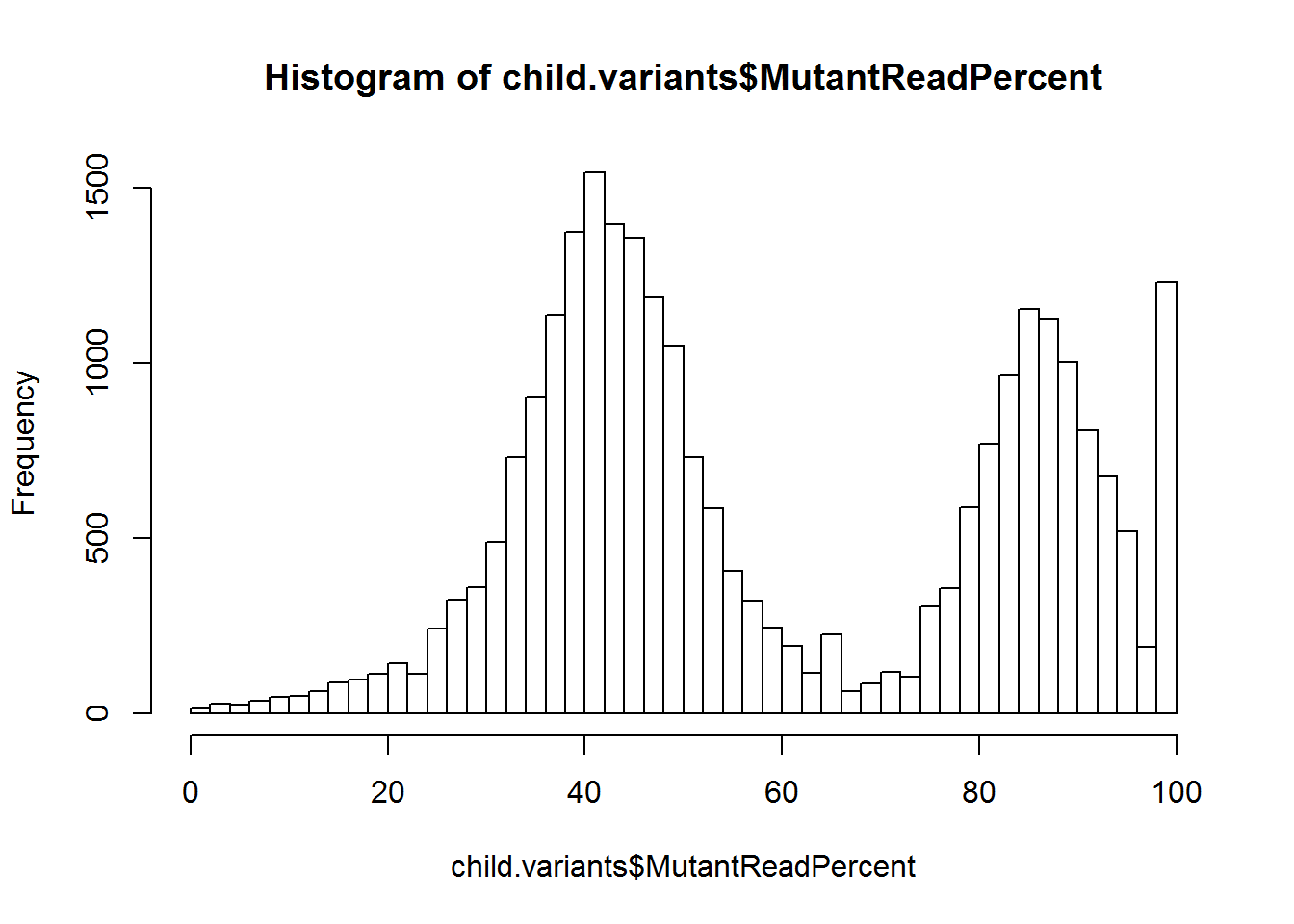• Plot a boxplot of the MutantReadPercent values from both the original child variants and the same column from the filtered dataset you made in Exercise 5 (MutantReadPercent>=70). Check that the distributions look different.

The boxplot function can take multiple vectors as input so we can simply pass the two vectors separtately. If we do this however the datasets will not be named (since the function doesn’t know what they are called). To fix this we would have to either explicitly set the names using the ‘names=’ parameter to the function. Alternatively we could put the two vectors into a list which would allow us to set the slot names for the list, and these will be picked up by boxplot automatically. Here we’ll do it both ways so you can see the options for how to do this.

boxplot(child.variants$MutantReadPercent, child.variants.filtered$MutantReadPercent, names=c("Original","Filtered"))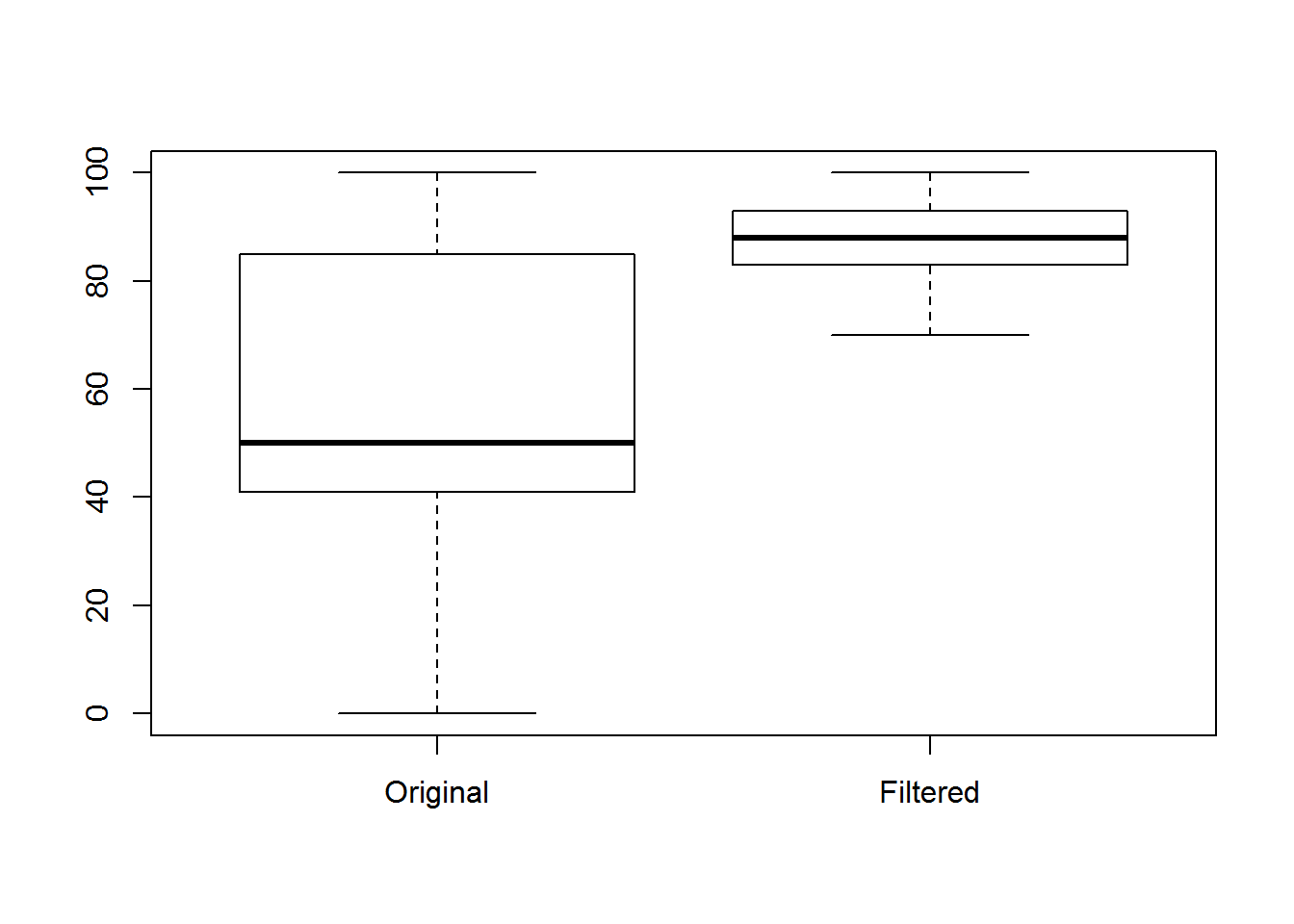boxplot(
list(
Original=child.variants$MutantReadPercent, Filtered=child.variants.filtered$MutantReadPercent
)
)• Plot the results of the vector created in Exercise 5 (‘mutation.counts’) as a barplot. Use the names.arg function to show which mutation is which and use the rainbow() function to give the bars different colours

In our case, because we put slot names onto our mutation.counts vector we don’t need to use names.arg as barplot will use the slot names by default. We’ll change the names later to show how you would do this anyway.

The rainbow() function needs to know how many colours to generate. We could just hard-code this since we know there are 4 values, but it’s generally nicer to calculate the value we need from the data. That way if we later want to change the number of mutatations we counted the code will still work.

barplot(mutation.counts, col=rainbow(length(mutation.counts)))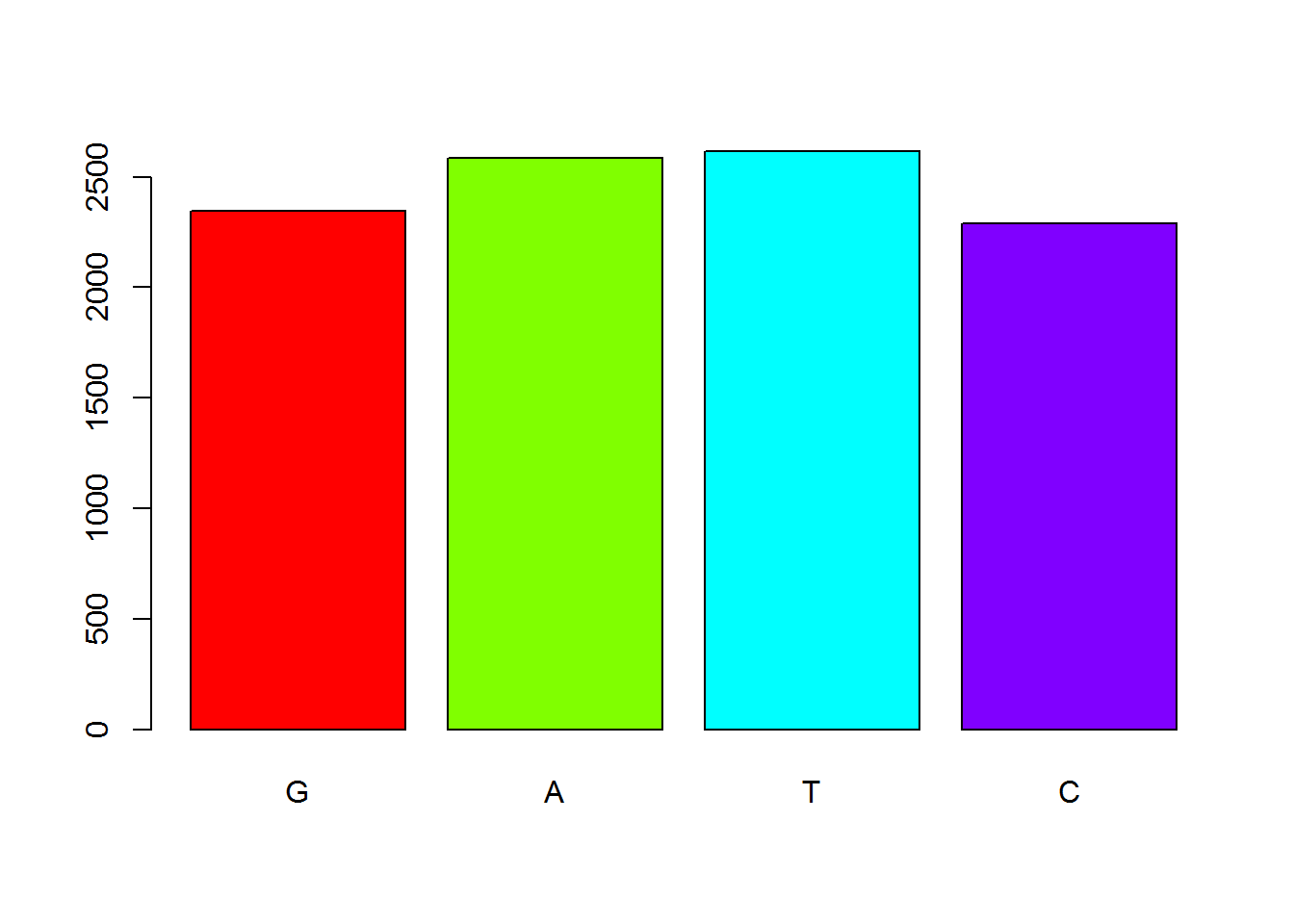If we did want to explicitly set the names then we could do that.

barplot(
mutation.counts,
col=rainbow(length(mutation.counts)),
)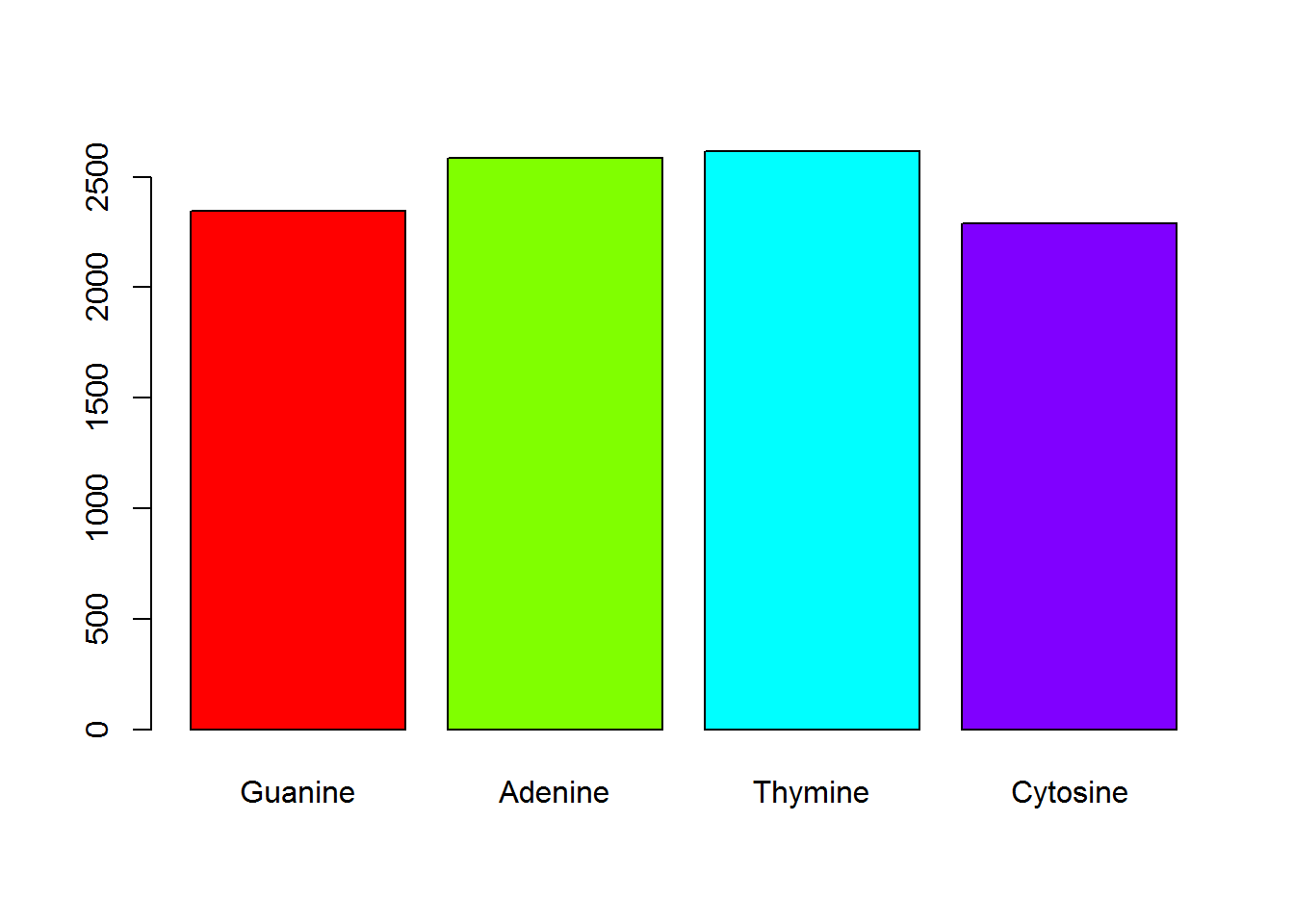## Exercise 7

• Read in the file’neutrophils.csv’. This is a comma-delimited file so use read.csv().
setwd("O:/Training/Introduction to R/R_intro_data_files")

head(neutrophils)
##        DMSO   TGX.221    PI103     Akt1
## 1 144.43930  99.61073 41.95241 111.8013
## 2 135.71670 115.35760 57.46430 124.1805
## 3  57.88828 106.44840 41.01954 126.7738
## 4  66.71269 115.89830 63.12587 130.9577
## 5  73.36981  75.96729       NA  88.6273
## 6  83.43180        NA       NA 147.8813
• Create a boxplot of the 4 samples and put a suitable title on the plot.

Since our data frame contains only the data we want to plot, and boxplot can take a list (which a data frame is) as input, we ca just pass the whole thing to the boxplot function.

boxplot(neutrophils, main="Range of values for different samples")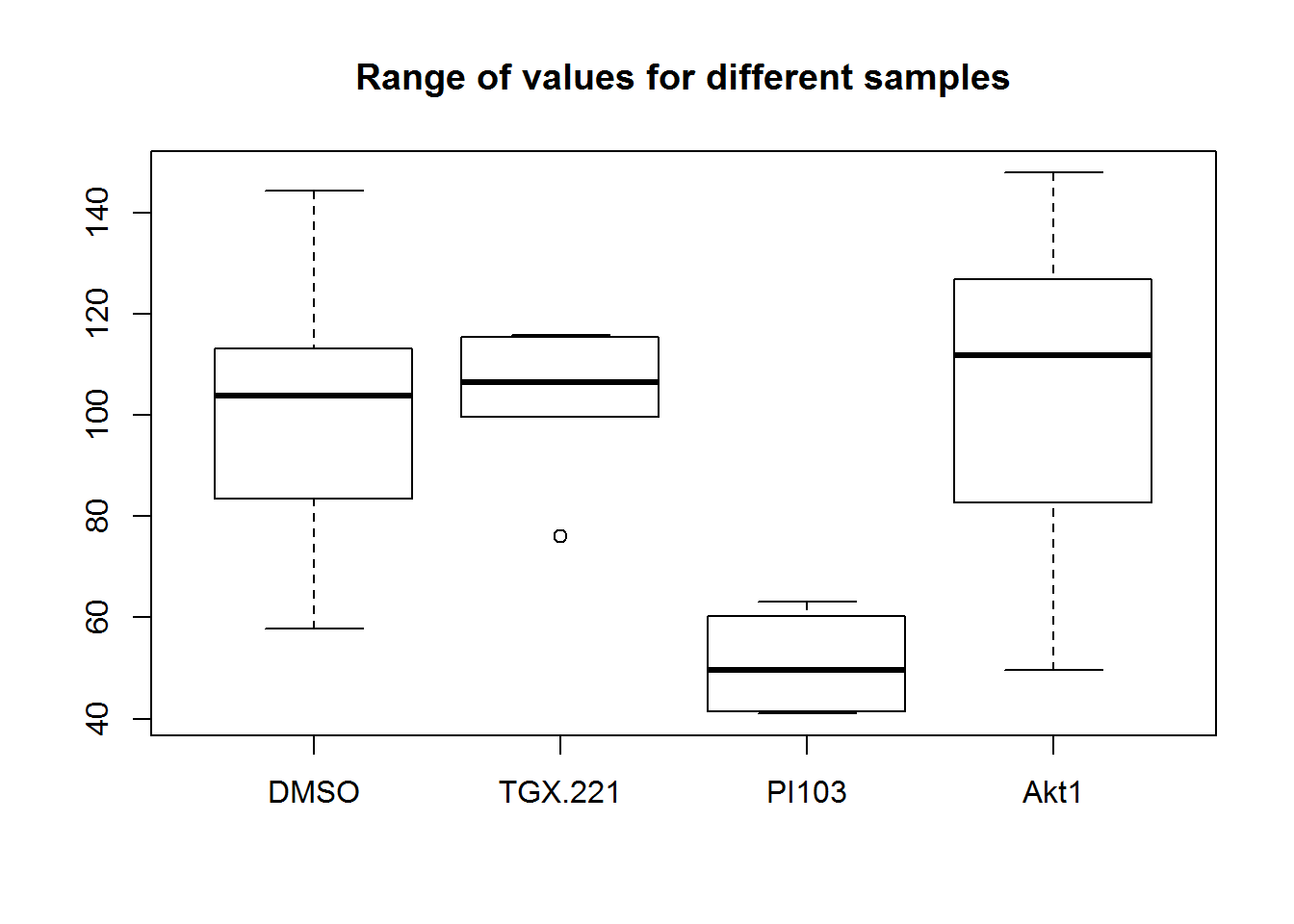• Use the colMeans() function to calculate the mean of each dataset. Plot the means as a barplot

If we try this on the data as it stands then we won’t get the answer we want, since there are missing (NA) values in our dataset.

colMeans(neutrophils)
##     DMSO  TGX.221    PI103     Akt1
## 100.8013       NA       NA       NA

To get colMeans to ignore the NA values when calculating the mean we can pass the na.rm=TRUE option.

colMeans(neutrophils, na.rm = TRUE) -> mean.values

mean.values
##      DMSO   TGX.221     PI103      Akt1
## 100.80126 102.65646  50.89053 103.91649

Finally we can plot these.

barplot(mean.values)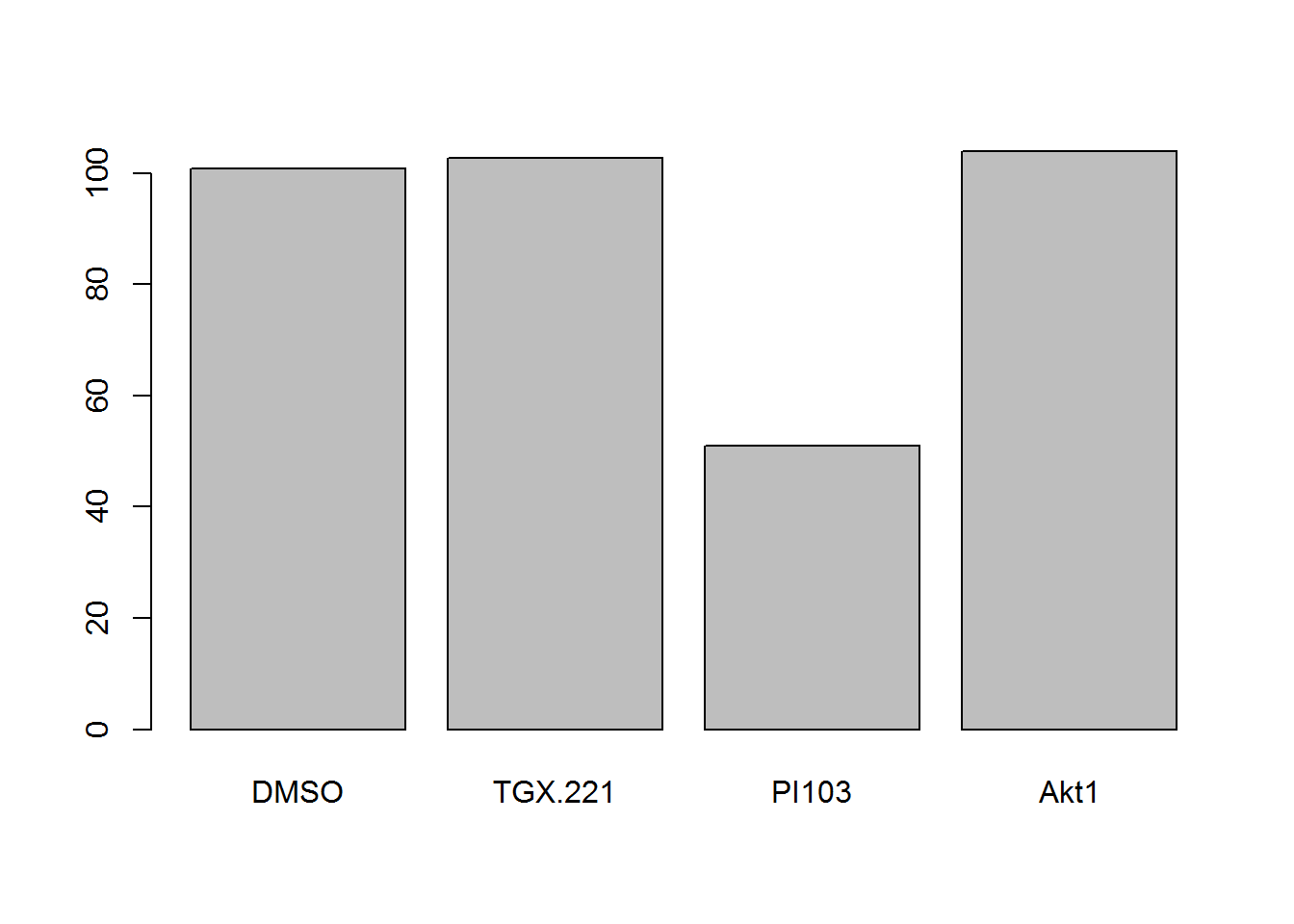• Read in the ‘brain_bodyweight.txt’ file. The first column contains species names, not data, so use row.names=1 to set these up correctly for your data frame.

This is a tab-delimited text file, so we’re using read.delim to read this in. In addition to the row.names parameter I’m also going to set check.names=FALSE so R doesn’t modify the column names (to remove spaces).

setwd("O:/Training/Introduction to R/R_intro_data_files")
read.delim("brain_bodyweight.txt", row.names=1, check.names = FALSE) -> brain.bodyweight

head(brain.bodyweight)
##                 Body weight (kg) Brain weight (g)
## Cow                       465.00            423.0
## Grey Wolf                  36.33            119.5
## Goat                       27.66            115.0
## Guinea Pig                  1.04              5.5
## Diplodocus              11700.00             50.0
## Asian Elephant           2547.00           4603.0

You can see that the species names have been correctly set as row names and that the column names are in tact.

• Log transform the data (base2)

Since all of the data frame is data we can simply pass the whole structure to the log2 function. Since we’re not going to use the untransformed data again we’ll overwrite the original data frame.

log2(brain.bodyweight) -> brain.bodyweight

head(brain.bodyweight)
##                 Body weight (kg) Brain weight (g)
## Cow                   8.86108691         8.724514
## Grey Wolf             5.18308946         6.900867
## Goat                  4.78972925         6.845490
## Guinea Pig            0.05658353         2.459432
## Diplodocus           13.51422091         5.643856
## Asian Elephant       11.31458324        12.168359
• Create a scatterplot with default parameters with the log transformed data.

We create a scatterplot with the plot() function. We need to pass the two vectors as the x and y values. Since they are in the correct order in our data frame we can, in this case, just pass the whole dataframe. This also has the advantage of setting the correct labels for the axes as they will be taken from the column names.

plot(brain.bodyweight)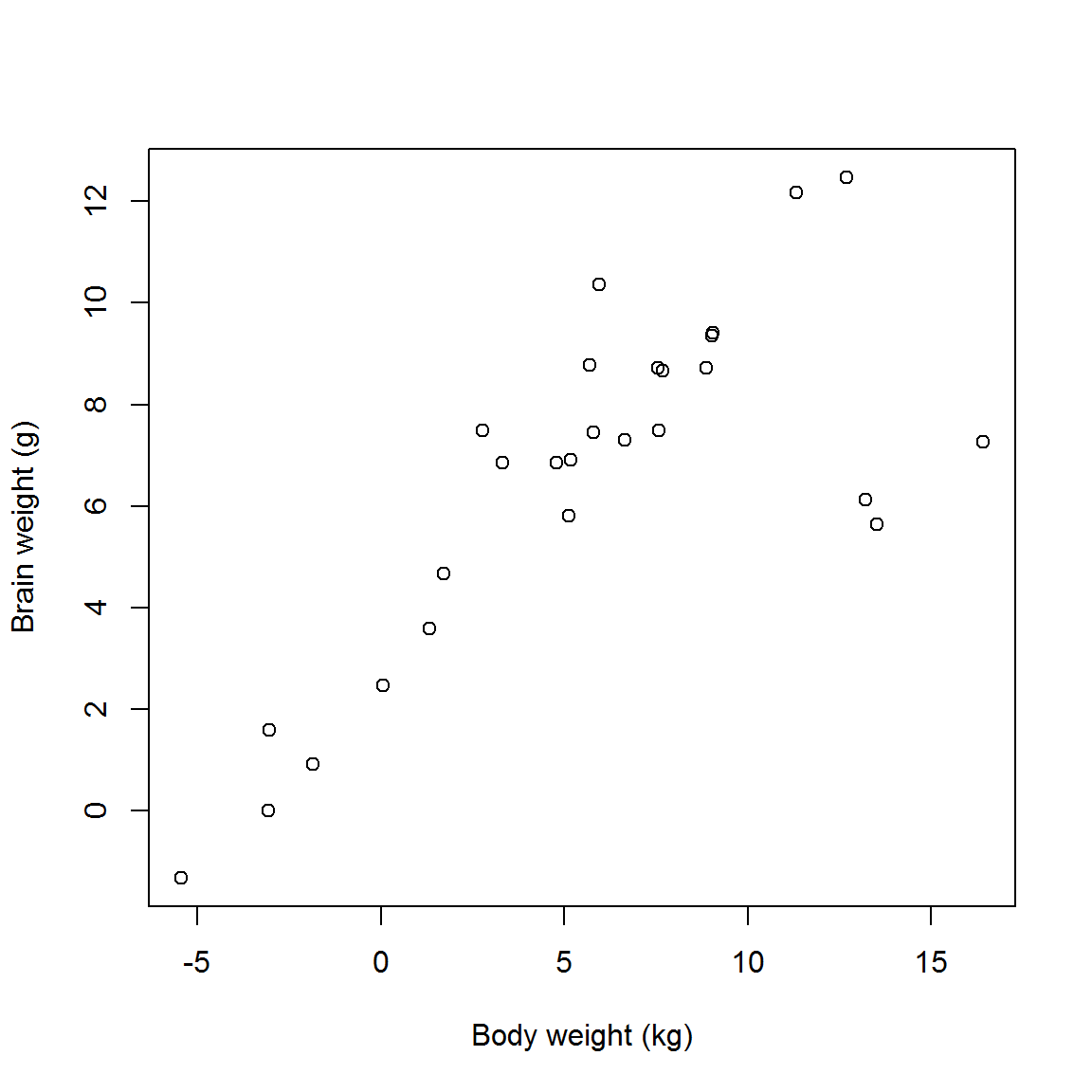• Create the same scatterplot but experiment with some parameters.
plot(
brain.bodyweight,
pch=19,
col="red",
cex=1.5,
main="Brainweight vs Bodyweight",
xlab="Bodyweight (log2 kg)",
ylab="Brainweight (log2 kg)"
)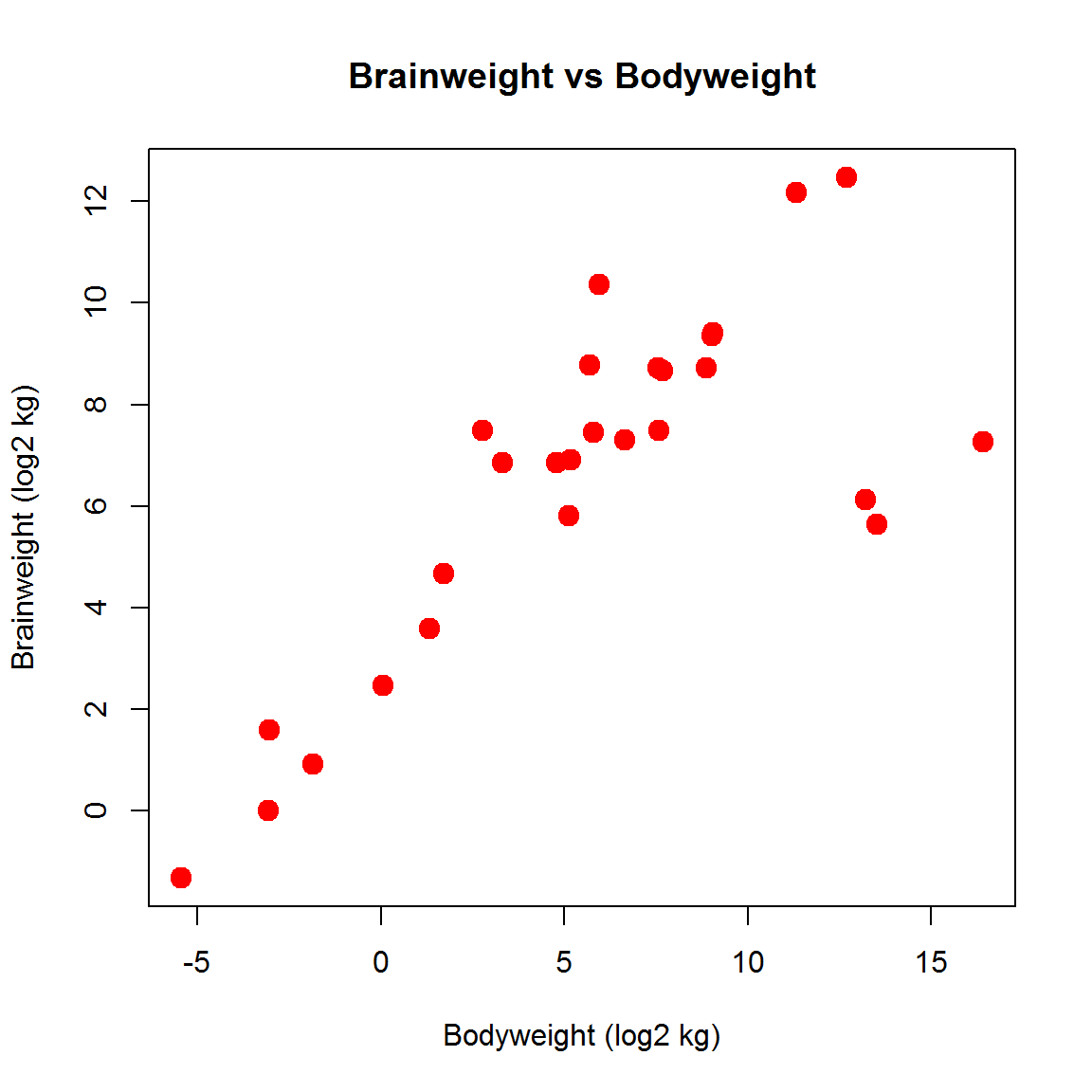• Read in the file chr_data.txt
setwd("O:/Training/Introduction to R/R_intro_data_files")

head(chr.data)
##   chr position GM06990_ABL1 GM06990_MLLT3
## 1   8        1  -0.04661052    -0.1816292
## 2   8   100001   0.25605089     0.4625507
## 3   8   200001   0.25605089    -0.1816292
## 4   8   300001   0.86137348    -0.5037192
## 5   8   400001   0.86137348    -0.5037192
## 6   8   500001   0.86137348    -0.5037192
• Remove the first column

This will involve a selection for the columns we want to keep, and then overwriting the original data with the selected subset. We could simply do a selection for columns 2,3 and 4.

head(chr.data[,2:4])
##   position GM06990_ABL1 GM06990_MLLT3
## 1        1  -0.04661052    -0.1816292
## 2   100001   0.25605089     0.4625507
## 3   200001   0.25605089    -0.1816292
## 4   300001   0.86137348    -0.5037192
## 5   400001   0.86137348    -0.5037192
## 6   500001   0.86137348    -0.5037192

We also also use a little trick, which is that if you use negative values for the index positions, you can say which positions you don’t want, instead of which ones you do want.

chr.data[,-1] -> chr.data
head(chr.data)
##   position GM06990_ABL1 GM06990_MLLT3
## 1        1  -0.04661052    -0.1816292
## 2   100001   0.25605089     0.4625507
## 3   200001   0.25605089    -0.1816292
## 4   300001   0.86137348    -0.5037192
## 5   400001   0.86137348    -0.5037192
## 6   500001   0.86137348    -0.5037192
• Draw a line graph of the position against the ABL1 dataset

For this we can use the plot() function with type=“l” (thats a the letter l rather than the number 1).

plot(
chr.data$position, chr.data$GM06990_ABL1,
type="l",
col="blue",
xlab="Chromosome Position (bp)",
ylab="z-score",
main="Z scores vs Genomic Position",
lwd=2
)

legend(
"topright",
"ABL1",
fill="blue"
)